| Technical Writer: Poornachandra Sarang | Technical Review: ABCOM Team | Copy Editor: Anushka Devasthale | Last Updated: July 20, 2020 | Level: Advanced |
Disclaimer: The purpose of this tutorial is to teach how to apply a multi-class classifier on a high-featured dataset. The model described here should not be used for cancer diagnosis.

When a doctor suspects that a patient may have cancer, probably the first thing he does is to collect his tumor sample. He then puts this sample through the genetic sequencing of DNA. A tumor can have thousands of genetic mutations. Briefly, a ‘mutation’ is a small change in a gene that may indicate the presence of cancer. An important aspect of such an analysis is that for every gene, there is a variation associated with it.

The process for cancer diagnosis is as follows:

1. A molecular pathologist gets a list of variations for the patient’s gene.
2. He searches the medical literature for these selected genetic variations.
3. He spends considerable time analyzing the evidence related to each of these variations.
4. Finally, he concludes with the type of cancer.

We will be developing a multi-class classification that will help the molecular pathologist to search the entire literature for his selected keywords (gene, variations, etc.) and provide him a smaller subset of the documents to study. In short, the model will help in narrowing down his searches on the entire literature to identify the keywords of his interest. Based on this, he will be able to conclude the type (class) of cancer which is going to be the target variable for our model training. So, let us learn how to apply the multi-class classification technique of machine learning to aid the doctor in his diagnosis of the cancer patient.

## Creating a Project

Create a Colab notebook and rename it to `CancerDiagnosis`. Import the following libraries. Do not know how to use Colab? Here is a short tutorial.

``````import pandas as pd
import matplotlib.pyplot as plt
import re
import warnings
import numpy as np
from sklearn.calibration import CalibratedClassifierCV
from sklearn.preprocessing import normalize
from sklearn.feature_extraction.text import CountVectorizer
import seaborn as sns
from sklearn.metrics import confusion_matrix
from sklearn.metrics.classification import  log_loss
from sklearn.linear_model import SGDClassifier
from scipy.sparse import hstack
from sklearn.model_selection import train_test_split
from sklearn.linear_model import LogisticRegression
``````

You can download the full dataset from Kaggle site. Optionally, you may download the two text files needed for our project from my GitHub. These files are the partial data taken from the Kaggle competition site.

``````!wget http://abcom.com/public-data/tutorials/ml-cancer-detection/training_text.zip

!unzip training_text.zip

!wget http://abcom.com/public-data/tutorials/ml-cancer-detection/training_variants.zip

!unzip training_variants.zip
``````

Examine the contents of the training_variants file.

``````data = pd.read_csv('/content/training_variants')
data
``````

The output is as follows :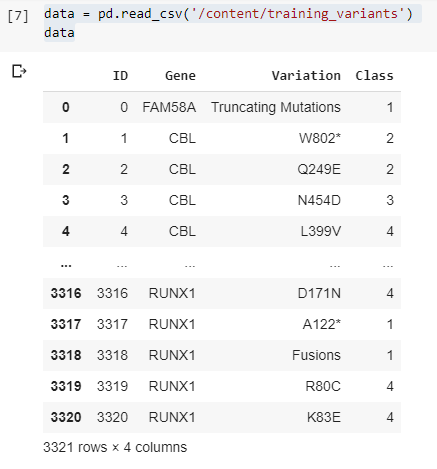As you can see, there are 3321 records, each containing four fields. For each Gene, variations and the corresponding class is listed. For example, we observe that a gene named `CBL` has four variations - `W802`, `Q249E`, `N454D`, and `L399V`. The associated class for these four variations is 2,2,3, and 4. These classes indicate the type of cancer. In the dataset, the classification is provided for 9 classes all of which do not indicate a presence of cancer. We leave the further introspection to the doctors; we will just provide them with the class number.

Next, load the contents of the training_text file.

``````data_text = pd.read_csv("/content/training_text", sep = "\|\|", engine = "python", names = ["ID","TEXT"], skiprows = 1)
``````

The output is as follows: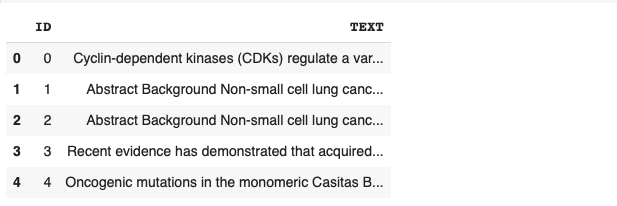As you can see, for each record (ID) in the training-variants datafile, some text related to cancer research is provided. For doctors, this text makes lots of sense in taking their decisions.

Let us examine one of the records:

``````data_text['TEXT']
``````

The output is as follows: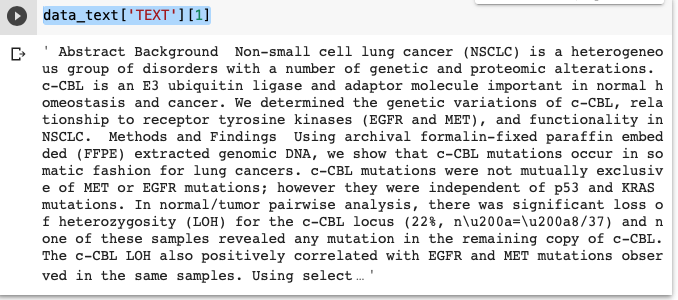Now, to give you some idea about how the doctors would use the above two files to diagnose a patient, I will take a single record from the above-created `data_text` and explain to you the process. Let us take the same data text displayed above and split it into a bag of words:

``````lu = data_text['TEXT'].split()
print(lu)
``````

The output is as follows: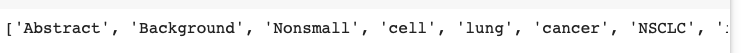So, we now have a bag of words from the original text item. Let us vectorize them using the `countVectorizer`. The `CountVectorizer` transforms a corpus of text to a matrix of token counts. We use the following code fragment for vectorization.

``````gene_vectorize = CountVectorizer()
train_gene_feature_onehotCodin = gene_vectorize.fit_transform(lu)
lop=gene_vectorize.vocabulary_
print(lop)
``````

The output is: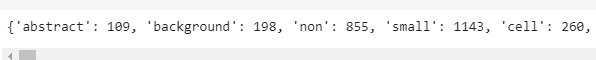As you can see, each word in our bag has some unique index associated with it.

Now, we can search this text for the presence of `gene` and `variation` from our training_variants datafile.

``````lst = []
for k in lop.keys():
if(k=="cbl" or k=="w802*" or k=="q249e" or k=="n454d"):
lst.append(k)
print(lst)
``````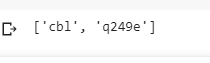In the above code, we check for the presence of `cbl`, `w802*`, and two more variations. Having found the desired text, we can look up cancer `class` from the training_variants file. This is how the doctors would use these two files to determine the type of cancer.

Now to provide the full search on all the text items, we will merge the two text files on the ID field:

``````result = pd.merge(data, data_text, on ='ID', how = 'left')
``````

As you can see, scanning all the text items would be a tremendous task for a human being. So, we will develop an ML model to provide us a multi-class classification on this dataset. Let us begin with Data Preprocessing.

## Pre-processing Data

To remove the digits and the special characters from the text, we define a function as follows:

``````import string
regex1 = re.compile('[%s]' % re.escape(string.digits))
regex2 = re.compile('[%s]' % re.escape(string.punctuation))
def remove(sentence):
reg = regex1.sub('',sentence)
reg = regex2.sub('',reg)
return reg
``````

Apply this function on the dataset:

``````data_text['TEXT'] = data_text['TEXT'].apply(lambda x: remove(str(x)))
``````

Replace all null values with `gene` and `variation`.

``````result.loc[result['TEXT'].isnull(),'TEXT'] = result['Gene'] +' '+result['Variation']
``````

Our data is pre-processed. We will now create training and testing datasets.

## Creating Datasets

We define our target variable as follows:

``````y_true = result['Class'].values
``````

Split the dataset into training and testing by maintaining the distribution of output variable `y_true`. This is achieved by setting the `stratify` parameter to `y_true`.

``````X_train, X_test, Y_train, Y_test = train_test_split(result,
y_true,
stratify = y_true,
test_size = 0.2)
``````

Likewise, create a cross-validation dataset.

``````X_train_cv, X_test_cv, Y_train_cv, Y_test_cv = train_test_split(X_train, Y_train,
stratify = Y_train, test_size = 0.2)
``````

## Model Choice

From the dataset that we have, you easily understand that it is a high dimensionality data with several keywords in the text acting as features and the 9 class values as output targets. As this is a multi-class classification problem, we will use logistic regression with class balancing as our model choice. For the model training, we will use the Log Loss (Logarithmic Loss) as a performance measurement function. In Log Loss the prediction input is a probability value between 0 and 1. The goal is to minimize this value, with a log loss value of 0 indicating a perfect model. If you are curious about the mathematical model behind this, the logarithmic loss function Fi is written with the following formula.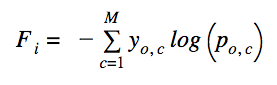Where summation of log loss values is taken across all classes. The `yo,c` is the binary variable with expected values and `po,c` is the classification probability output for the `oth` instance and the `cth` label. To have a benchmark value for our model’s performance, we will create some random data and generate a log-loss. Then, we will calculate the log-loss on our training and test data. If the log-loss on our test data shows improvement over the random data, then we can safely assume that our model is performing well and can be used on unseen data. So, let us first create some random data and a model based on it.

## Random Model for Benchmarking

We will generate 9 random numbers in the range 1 to 9 representing the 9 output classes that we want for this application. We will sum up these 9 numbers and divide each one by the sum to represent probabilities for each class. Note that the sum of all these probabilities would be one. We will pick the highest probability value to make the prediction and then plot a confusion matrix and also compute the total log-loss.

First, we define a function for plotting confusion matrix:

### Function for Confusion Matrix

In the case of multi-class classification, the confusion matrix will help provide a visual representation of the model’s performance. The matrix is a table, where rows define the actual values and columns define the predicted values. If all diagonal cells of the matrix have high values, the model is considered to have a good performance.

We define the function for plotting a confusion matrix as follows:

``````def plot_confusion_matrix(test_y, predict_y):
C = confusion_matrix(test_y, predict_y)

labels = [1,2,3,4,5,6,7,8,9]
# representing in heatmap format
print("-"*20, "Confusion matrix", "-"*20)
plt.figure(figsize = (20,7))
sns.heatmap(C, annot = True, cmap = "YlGnBu", fmt = ".3f",
xticklabels = labels, yticklabels = labels)
plt.xlabel('Predicted Class')
plt.ylabel('Original Class')
plt.show()
``````

We will now create some random target values for the test dataset that we created earlier and use the above function to plot the confusion matrix and print its log-loss value.

### Creating Random Model

The following code generates some random values for the output classes and assigns them to each row of the test dataset. It then computes the log loss between the actual and predicted values and prints its value.

``````# Test dataset
test_data_len = X_test.shape
# Number of rows equals number of test data points
test_predicted_y = np.zeros((test_data_len,9))
for i in range(test_data_len):
# generate 9 random  numbers for our random classes.
rand_probs = np.random.rand(1,9)
# divide each with the sum of all
test_predicted_y[i] = ((rand_probs/sum(sum(rand_probs))))
print("Log loss on Test Data using Random Model",
log_loss(Y_test, test_predicted_y, eps=1e-15))
``````

The output is as follows:
`Log loss on Test Data using Random Model 2.5496030675752293`
Note that the output will vary on each run due to the randomness of the input data. Finally, we plot a confusion matrix representing all 9 classes.

``````# get the max probability
predicted_y = np.argmax(test_predicted_y, axis = 1)
plot_confusion_matrix(Y_test, predicted_y+1)
``````

The output is as follows: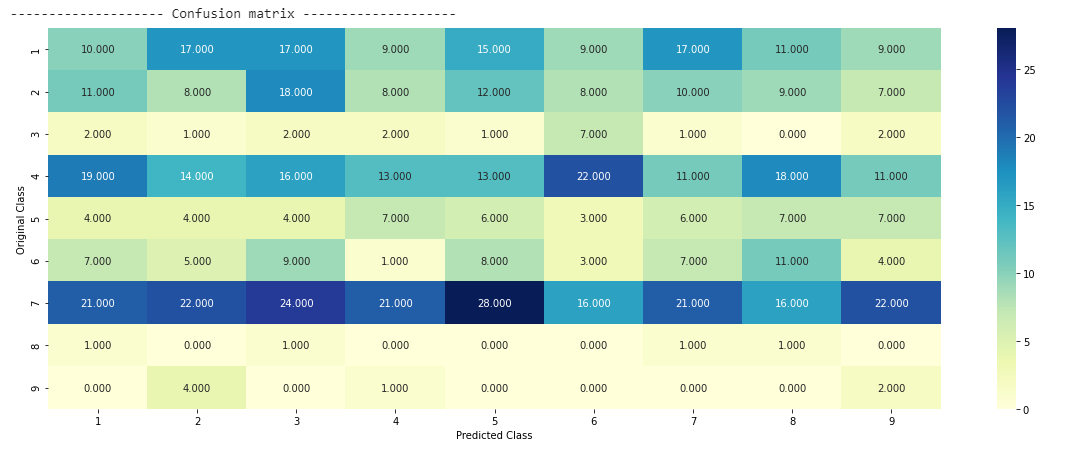In the above confusion matrix, you see that three are 10 data points for class 1 where the original and the predicted classes match. There are 8 data points for class 2 for which both original and predicted classes match, and so on. Thus, using the confusion matrix, you get a better visualization of the model’s performance than looking at a single value of log loss. As you are aware, if all diagonal values are high, the model is considered to be best tuned.

Just the way you printed the log loss on a test data, you could do the same on the cross-validation data using the following code:

``````cv_data_len = X_test_cv.shape

# Cross validation dataset
cv_predicted_y = np.zeros((cv_data_len,9))
for i in range(cv_data_len):
rand_probs = np.random.rand(1,9)
cv_predicted_y[i] = ((rand_probs/sum(sum(rand_probs))))

print("Log loss on Cross Validation Data using Random Model",:
``````

Output:
`Log loss on Cross Validation Data using Random Model 2.5192218893929446`

## Encoding Text

For model fitting, we need to convert our textual features into numerical values. We will use Bag-of-Words (BOW) for tokenization and use one-hot encoding on the tokens.

We encode the `gene` using the following code:

``````gene_vectorizer = CountVectorizer()
train_gene_feature_onehotCoding = gene_vectorizer.fit_transform(X_train['Gene'])
test_gene_feature_onehotCoding = gene_vectorizer.transform(X_test['Gene'])
cv_gene_feature_onehotCoding = gene_vectorizer.transform(X_train_cv['Gene'])
``````

Note that the above code encodes gene features in all three datasets. Likewise, we encode variation features using the following code:

``````# one-hot encoding of variation feature.
variation_vectorizer = CountVectorizer()
train_variation_feature_onehotCoding = variation_vectorizer.fit_transform(X_train['Variation'])
test_variation_feature_onehotCoding = variation_vectorizer.transform(X_test['Variation'])
cv_variation_feature_onehotCoding = variation_vectorizer.transform(X_train_cv['Variation'])
``````

Lastly, we encode the text feature using the code given below. In the CountVectorizer constructor, we specify the minimum frequency of occurrence to be 3 and discard all English stop words. We do this for text features only as the text feature has lots of words while the gene and variation have only one word in it. If we do not restrict the tokenization to words having a minimum frequency of 3, then the number of features for training would turn out to be very large and unmanageable in terms of required resources for training. Also, it may result in overfitting. We also normalize each word as in case of the text feature a particular word may have a high frequency. In the case of gene and variation, most of the values were 0 and the max value was 1, so normalization was not required in those cases.

``````# one-hot encoding of text feature.
# Minimum frequency for words = 3 and remove all stop words
text_vectorizer = CountVectorizer(min_df = 3, stop_words = 'english')
train_text_feature_onehotCoding = text_vectorizer.fit_transform(X_train['TEXT'])
train_text_feature_onehotCoding = normalize(train_text_feature_onehotCoding, axis = 0)
test_text_feature_onehotCoding = text_vectorizer.transform(X_test['TEXT'])
test_text_feature_onehotCoding = normalize(test_text_feature_onehotCoding, axis = 0)
cv_text_feature_onehotCoding = text_vectorizer.transform(X_train_cv['TEXT'])
cv_text_feature_onehotCoding = normalize(cv_text_feature_onehotCoding, axis = 0)
``````

### Merging Features

For our analysis, all features - gene, variation, and text are equally important. So we merge them in a single list before feeding it to our logistic regression algorithm. We use the hstack function to stack the various data points and finally convert them into numpy arrays for inputting them to our model.

``````# merging gene, variation and text features
train_gene_var_onehotCoding = hstack((train_gene_feature_onehotCoding,
train_variation_feature_onehotCoding))
test_gene_var_onehotCoding = hstack((test_gene_feature_onehotCoding,
test_variation_feature_onehotCoding))
cv_gene_var_onehotCoding = hstack((cv_gene_feature_onehotCoding,
cv_variation_feature_onehotCoding))

train_x_onehotCoding = hstack((train_gene_var_onehotCoding,
train_text_feature_onehotCoding)).tocsr()
train_y = np.array(list(X_train['Class']))

test_x_onehotCoding = hstack((test_gene_var_onehotCoding,
test_text_feature_onehotCoding)).tocsr()
test_y = np.array(list(X_test['Class']))

cv_x_onehotCoding = hstack((cv_gene_var_onehotCoding,
cv_text_feature_onehotCoding)).tocsr()
cv_y = np.array(list(X_train_cv['Class']))
``````

Now, we are ready to build our model.

## Logistic Regression with Class Balancing

As mentioned before, we will use logistic regression for modeling our dataset. The logistic regression performs well for high dimension data and it is also interpretable. First, we will identify the best alpha value for training the model.

### Selecting Best Alpha for Model Training

The model training code is shown here. After the code, I will explain the relevant statements.

``````alpha = [10 ** x for x in range(-6, 3)]
cv_log_error_array = []
for i in alpha:
print("for alpha =", i)
clf = SGDClassifier(class_weight='balanced',
alpha = i, penalty = 'l2',
loss = 'log', random_state = 42)
clf.fit(train_x_onehotCoding, train_y)
sig_clf = CalibratedClassifierCV(clf, method = "sigmoid")
sig_clf.fit(train_x_onehotCoding, train_y)
sig_clf_probs = sig_clf.predict_proba(cv_x_onehotCoding)
cv_log_error_array.append(log_loss(cv_y, sig_clf_probs,
labels = clf.classes_, eps = 1e-15))
print("Log Loss :",log_loss(cv_y, sig_clf_probs))
``````

We use the `SGDClassifier` function for logistic regression. The `class_weight` parameter is set to balanced so that it can take care of an imbalance dataset. In our dataset, some classes occur more frequently than others. We set the l2 regularization to avoid overfitting. The loss parameter is set to log, indicating that we want to apply logistic regression. Also, we use the calibrated classifier that predicts the class probabilities rather than the absolute values. Predicting probabilities provides more nuanced ways to evaluate the model’s skills. Running the code gives the following output:
```for alpha = 1e-06 Log Loss : 0.800011752988093 for alpha = 1e-06 Log Loss : 0.817652656156739 for alpha = 1e-05 Log Loss : 0.7239067948570755 for alpha = 0.0001 Log Loss : 0.43229795092997625 for alpha = 0.001 Log Loss : 0.48921806403913265 for alpha = 0.01 Log Loss : 0.7149303850764087 for alpha = 0.1 Log Loss : 1.3211992535744743 for alpha = 1 Log Loss : 1.5850326732031788 for alpha = 10 Log Loss : 1.6174113158356398 for alpha = 100 Log Loss : 1.6208749923611223```

Now, we need to select the best alpha value and redo the training with it.

### Train with Best Alpha

We select the best alpha value by using `argmin` function and then redo the training using the selected alpha value.

``````best_alpha = np.argmin(cv_log_error_array)
clf = SGDClassifier(class_weight = 'balanced',
alpha = alpha[best_alpha], penalty = 'l2',
loss='log', random_state = 42)
clf.fit(train_x_onehotCoding, train_y)
sig_clf = CalibratedClassifierCV(clf, method = "sigmoid")
sig_clf.fit(train_x_onehotCoding, train_y)
``````

### Inference

After the training is over, we do inference on the datasets.

``````predict_y = sig_clf.predict_proba(train_x_onehotCoding)
print("The train log loss is:",
log_loss(Y_train, predict_y, labels = clf.classes_, eps = 1e-15))
predict_y = sig_clf.predict_proba(cv_x_onehotCoding)
print("The cross validation log loss is:",
log_loss(Y_train_cv, predict_y, labels = clf.classes_, eps = 1e-15))
predict_y = sig_clf.predict_proba(test_x_onehotCoding)
print("The test log loss is:",
log_loss(Y_test, predict_y, labels = clf.classes_, eps = 1e-15))
``````

You will see the following output:

```The train log loss is: 0.4577097974816002 The cross validation log loss is: 0.43229795092997625 The test log loss is: 1.100231148477779```

Compare these values with our earlier results of the random model, which are reproduced below for your quick reference.
```Log loss on Cross Validation Data using Random Model 2.5192218893929446 Log loss on Test Data using Random Model 2.5496030675752293```

As you can see, in both cases of validation and test datasets, the log-loss is reduced considerably from about 2.5 to less than 1.0. Thus, we can conclude that our model is performing well.

Now, we will do some model testing with the best hyperparameters that we have obtained above.

## Model Evaluation

For model testing and plotting the confusion matrix on the results we define the following function:

``````def predict_and_plot_confusion_matrix(train_x, train_y,
test_x, test_y, clf):
clf.fit(train_x, train_y)
sig_clf = CalibratedClassifierCV(clf, method = "sigmoid")
sig_clf.fit(train_x, train_y)
pred_y = sig_clf.predict(test_x)

# Display number of data points that are misclassified
print("Number of mis-classified points :",
np.count_nonzero((pred_y- test_y))/test_y.shape)
plot_confusion_matrix(test_y, pred_y)
``````

We pass the classifier instance in the above method, train the model with the training dataset and predict value on the test dataset. We print the number of misclassified points and the confusion matrix on the terminal.

The above method itself is called by first creating the classifier instance with the best alpha parameter.

``````clf = SGDClassifier(class_weight = 'balanced',
alpha = alpha[best_alpha], penalty = 'l2',
loss = 'log', random_state = 42)
predict_and_plot_confusion_matrix(train_x_onehotCoding,
train_y, cv_x_onehotCoding,
cv_y, clf)
``````

The output is as follows: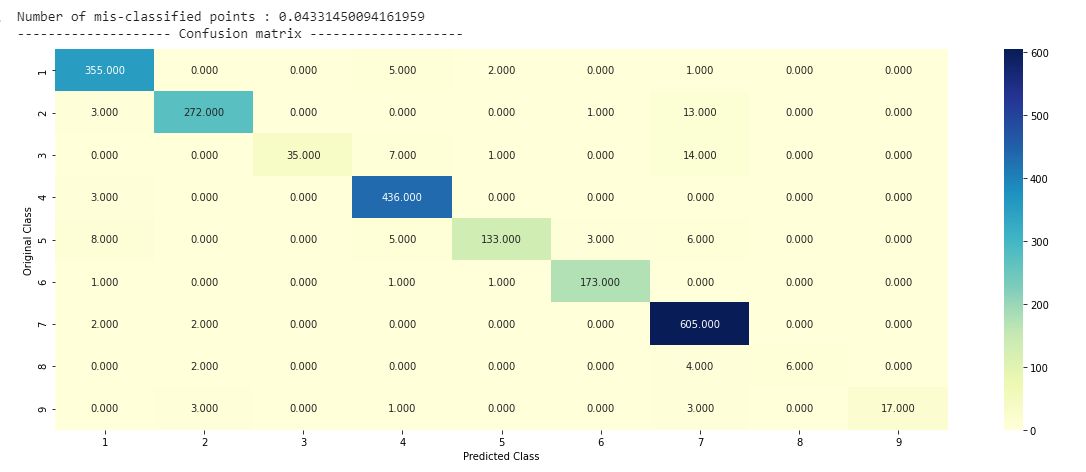As you can see, the number of misclassified points is just 4.3%. The confusion matrix shows most of the diagonal cells have high values. Thus, our model is doing very well.

Now, we are going to put our trained model for some real use.

## Real Use by Doctors

Consider that a doctor sees a patient with a tumor. He samples the tumor and gets the gene and variation details on the sample. Then he uses our model to find out the cancer class (a number in the range 1 to 9) and also the number of places in the text where the important keywords (features) are found. Based on this information, he does further diagnosis on the patient’s data. To demonstrate this use, I will pick up a record from our test dataset that gives us the gene and its variations. To determine the detected features in the dataset, we first write a small function called `getImportantFeatures` as follows:

``````def getImportantFeatures(indices, gene, variation, text, noOfFeatures):
gene_features = gene_vectorizer.get_feature_names()
variation_features = variation_vectorizer.get_feature_names()
text_features = text_vectorizer.get_feature_names()

gene_feat_len = len(gene_features)
var_feat_len = len(variation_features)
text_features_len =len(text_features)

word_present = 0
for i, v in enumerate(indices):
if v < gene_feat_len:
word = gene_features[v]
if word == gene:
word_present += 1
print("{}st Gene feature [{}] is present in query point [{}]".format(i+1, word,bool_var))

elif (v < gene_feat_len + var_feat_len):
word = variation_features[v - gene_feat_len]
if word == variation:
word_present += 1
print("{}th Variation feature [{}] is present in query point".format(i+1, word))
else:
word = text_features[v - (gene_feat_len + var_feat_len)]

if word in text.split():
word_present += 1
print("{}th Text feature [{}] is present in query point".format(i+1, word))

print("-"*63)
print("Out of the top "+str(noOfFeatures)+" features "+
str(word_present)+" are present in query point")
print("-"*63)
``````

The function simply looks for the gene, variation and top text features and prints the index values of the text items where those are found. Let us now take a sample data point, say at index 50, and print the analysis results given by our model. This is done using the following code:

``````testDataPoint = 50
top_features = 1000
predicted_cls = sig_clf.predict(test_x_onehotCoding[testDataPoint])
print("Predicted Class :", predicted_cls)
print("Predicted Class Probabilities:", np.round(sig_clf.predict_proba(test_x_onehotCoding[testDataPoint]),4))
print("Actual Class :", test_y[testDataPoint])
indices = np.argsort(-1*abs(clf.coef_))[predicted_cls-1][:,:top_features]
getImportantFeatures(indices, X_test.iloc[testDataPoint]["Gene"],
X_test.iloc[testDataPoint]["Variation"],
X_test.iloc[testDataPoint]["TEXT"], top_features)
``````

The output is as follows: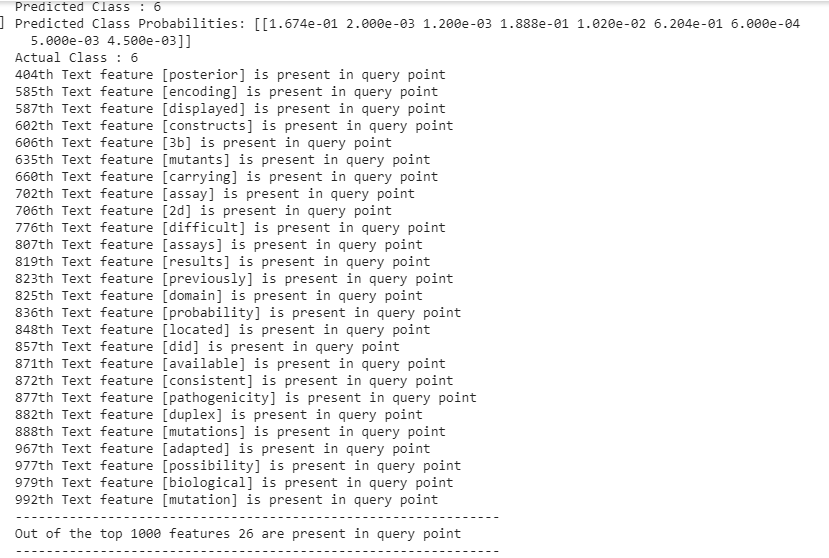From the above output, you can see that the predicted class is 6, with the highest probability of 0.6204 amongst all the other classes. This suggests the doctor that the probability of getting cancer in class 6 is about 62%. The actual class as known from our dataset too is 6. Note that in the real world situation, this actual class is not available to the doctor. The output also shows that 26 features are located in the literature text. For example, the words “posterior” and “encoding” are present in the text at index 404 and 585 respectively. The doctor can now study the text at these indices to further firm up his decision. You can easily make out that the doctor is now saving his tremendous valuable time in going through all the texts in the dataset and focusing only on those text items pointed out in the prediction output. You may test the model with another index value (the test datapoint) and see the results for yourself.

Finally, to make the model useful to the doctors in diagnosing unseen cases, you will need to provide an application-level user interface to the doctor where he enters the three fields - gene, variations, and some text of his own. This would create a record similar to the test record at index 50. The new record has to undergo the entire preprocessing that we did on our test dataset. Feed the pre-processed record to the predict method and wow it will spill out the predictions indicating the probable type of cancer class and some text to read to further up the claim.

## Conclusion

In this tutorial, you learned how to apply a multi-class classification using logistic regression to help the doctors diagnose a probable cancer patient. We used the SGDClassifier of sklearn and trained it for classifying 9 cancer types. You used the data supplied in the Kaggle competition for training the model. The use of the confusion matrix helped us in visualizing the model’s performance for a multi-class problem. You may now use the technique that you learned here in solving other multi-class classification problems with a large number of features and targets.